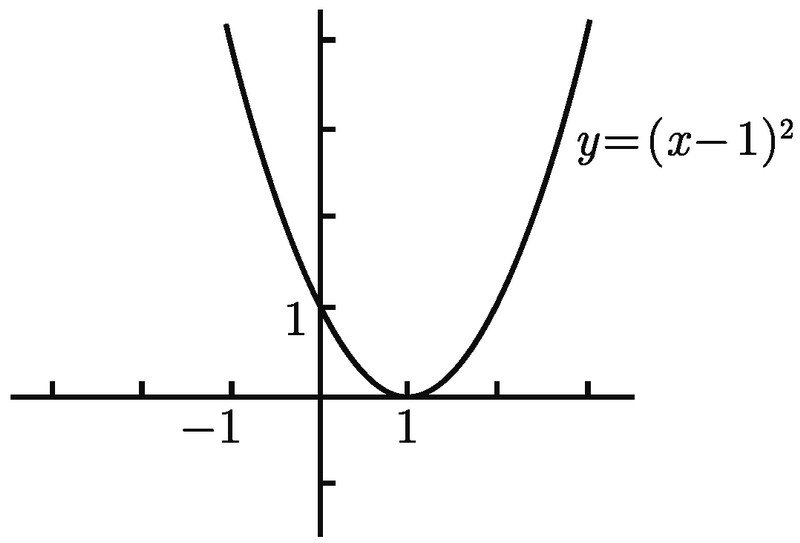# 普林斯顿微积分读本（修订版）(11)：函数、图像和直线 1.3(函数的复合)

$f(x)=g(h(j(x)))=g(h(2x-1))=g(5(2x-1)^4)=2^{5(2x-1)^4}$

$f(x)=\frac{1}{\tan(5\log_2(x+3))}.$

$f(x)=m(k(j(h(g(x))))).$

$f=m\circ k\circ j\circ h\circ g.$

$f=m\circ k\circ n\circ g.$

$j(x)=g(h(x))=g(\sin(x))=(\sin(x))^2$

j(x) = sin2(x) 比较一下. 函数 j 完全不同于乘积 x2 sin(x), 它同样不同于函数 k = hg. 函数 k 也是 gh 的复合函数, 不过是按另一个顺序的复合：

$k(x)=h(g(x))=h(x^2)=\sin(x^2).$

k 是另一个完全不同的函数. 这个例子说明, 函数的乘积和复合是不同的, 且函数的复合与函数顺序有关系, 而函数的乘积与函数顺序无关.